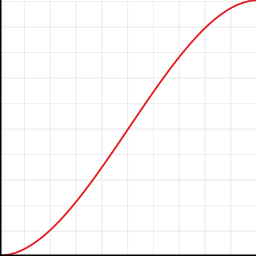### Interpolator

Realtime interpolation equation WYSIWYG editor

Use JavaScript syntax to create complex equations.

Result of equation is evaluated as y (depends on type of visualization: movement = vertical position from X to Y point; scaling = scale X and Y size from 0% to 100%; rotation = degress from 0° to 360°; alpha = opacity from 0% to 100%). Graph can show show y min. -1.0 and max. 2.0

Available input variables: x = time, d = duration

You can use all JavaScript Math functions/constants - see list here.
Use JS math functions without Math.!

#### Keyboard shortcuts

Use ALT+ or ALT+SHIFT+ (firefox) or CTRL+ALT+ (mac)

M - movement, S - scaling, R - rotation, A - alpha, X - stop

Sorry, your browser is not supported.

Equation (?):

Visualization:

Duration:

ms